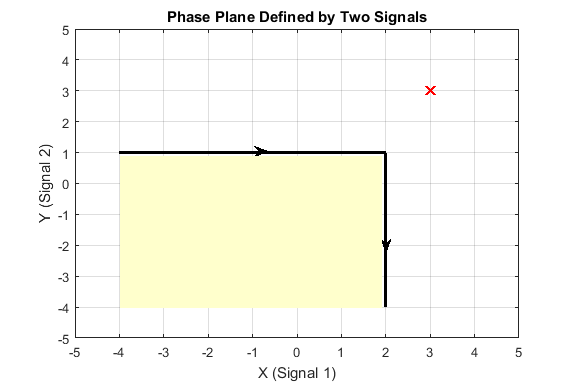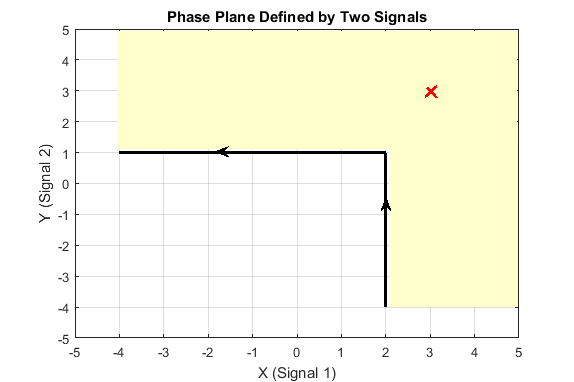# evalRequirement

Class: sdo.requirements.PhasePlaneRegion
Package: sdo.requirements

Evaluate satisfaction of piecewise-linear bound on phase plane trajectory of two signals

## Syntax

```evaluation = evalRequirement(requirement,signal1,signal2) evaluation = evalRequirement(requirement,signals) ```

## Description

`evaluation = evalRequirement(requirement,signal1,signal2)` evaluates whether the phase plane trajectory of the two signals `signal1` and `signal2` satisfies the piecewise-linear bound specified in the `requirement` object. The phase plane trajectory is a plot of the two signals against each other. A positive `evaluation` value indicates that the requirement has been violated.

`evaluation = evalRequirement(requirement,signals)` specifies the two signals as an n-by-2 array. The first column corresponds to the first signal, and the second column corresponds to the second signal. `n` is the number of time points in the signals.

## Input Arguments

expand all

Phase plane region requirement, specified as an `sdo.requirements.PhasePlaneRegion` object. In the object, you specify the piecewise-linear bounding edges.

Signals to be evaluated, specified as `timeseries` objects.

`signal1` corresponds to the `x`-value of the phase plane trajectory, and `signal2` corresponds to the `y`-value of the phase plane trajectory.

Signals to be evaluated, specified as an n-by-2 array. The first column corresponds to the first signal, the `x`-value of the phase plane trajectory. The second column corresponds to the second signal, the `y`-value of the phase plane trajectory. `n` is the number of time points in the signals.

## Output Arguments

expand all

Evaluation of the phase plane region requirement, returned as a scalar value. The software finds the trajectory point that is closest to the bounding region and then calculates `evaluation` as a scaled distance between this point and the closest bound edge. A positive value indicates that the requirement has been violated and some or all the trajectory points defined by the two test signals lie outside the specified bounding region. A negative value or `0` indicates that the requirement has been satisfied. When `evaluation` is `0`, the closest trajectory point lies on the edge.

## Examples

expand all

Create a default requirement object.

`Requirement = sdo.requirements.PhasePlaneRegion;`

The requirement object specifies the bounding region as a single edge extending from (-1,0) to (-1,1) in the phase plane defined by two signals. `Requirement.Type` has the default value of `'<='`. This value implies the area to the left of the edge is out of bounds, where the forward direction is the direction of creation of the edge.

Specify the test signal data as `timeseries` objects.

```signal1 = timeseries(1-exp(-(0:10)')); signal2 = timeseries(sin((0:10)'));```

Evaluate the requirement.

`Evaluation = evalRequirement(Requirement,signal1,signal2)`
```Evaluation = -0.2632 ```

A negative value indicates that the requirement is satisfied, and the phase plane trajectory of the two test signal data is in the bounding region.

Create a requirement object to specify a piecewise-linear bound on the phase plane trajectory of two signals. The bound has two edges. The first edge extends from (-4,1) to (2,1). The second edge extends from (2,1) to (2,-4).

```Requirement = sdo.requirements.PhasePlaneRegion('BoundX',[-4 2; 2 2],... 'BoundY',[1 1; 1 -4]);```

Specify the bound type as `'>='`.

`Requirement.Type = '>=';`

The plot below shows the bounding edges in black. The arrows indicate the direction in which the edges were specified. When you specify the `Type` property as `'>='`, the out-of-bound area is always to the right of each edge, where the forward direction is the direction of creation of the edge. As a result, the out-of bound region is the yellow shaded area, and a trajectory point located at (3,3) is in the bounded region.Evaluate the requirement for the phase plane trajectory point located at (3,3).

`Evaluation = evalRequirement(Requirement,[3 3])`
```Evaluation = -0.6389 ```

`evalRequirement` returns a negative number, indicating the requirement is satisfied.

Now create the requirement by changing the order of specification of the edges.

`set(Requirement,'BoundX',[2 2; 2 -4],'BoundY',[-4 1;1 1]);`The plot shows that the edges were created in the opposite order. So, even though the requirement type is still `'>='`, the out-of-bound region, which is always to the right of the edges, is now flipped.

Evaluate the requirement.

`Evaluation = evalRequirement(Requirement,[3 3])`
```Evaluation = 0.1087 ```

A positive `Evaluation` value indicates the requirement has been violated. Thus, for the same requirement type, the trajectory point at (3,3) is out of bounds when the edges are defined in the reverse order.

#### Introduced in R2016b